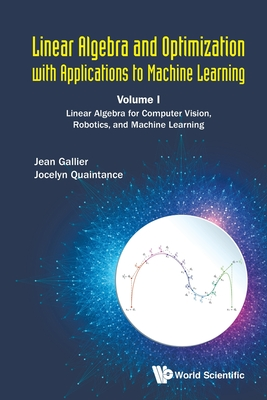### Linear Algebra and Optimization with Applications to Machine Learning:Volume I: Linear Algebra for Computer Vision, Robotics, and Machine Learning 线性代数和最优化在机器学习中的应用，卷I：用于计算机视觉，机器人和机器学习的线性代数计算机科学技术基础学科

1043.00

2020年01月22日

ＩＳＢＮ
9789811207716

550

• 图书详情
• 目次
• 买家须知
• 书评（0）
• 权威书评（0）

This book provides the mathematical fundamentals of linear algebra to practicers in computer vision, machine learning, robotics, applied mathematics, and electrical engineering. By only assuming a knowledge of calculus, the authors develop, in a rigorous yet down to earth manner, the mathematical theory behind concepts such as: vectors spaces, bases, linear maps, duality, Hermitian spaces, the spectral theorems, SVD, and the primary decomposition theorem. At all times, pertinent real-world applications are provided. This book includes the mathematical explanations for the tools used which we believe that is adequate for computer scientists, engineers and mathematicians who really want to do serious research and make significant contributions in their respective fields. Key Features: oThis book fills a gap in the market in that it is a mathematically rigorous book which provides topical applications for the fields of machine learning, computer vision, and robotics oThis book covers, in more depth than usual, duality (Chapter 9), vector and matrix norms (Chapter 7), and the spectral theorems (Chapter 15) oThis book will contain classroom tested exercises Professor Gallier has successfully used throughout his many years of teaching CIS 515: Fundamentals of Linear Algebra and Optimization. These multi-faceted exercises contain both mathematical proofs and computer programming

• 上一个
• 下一个
•
•